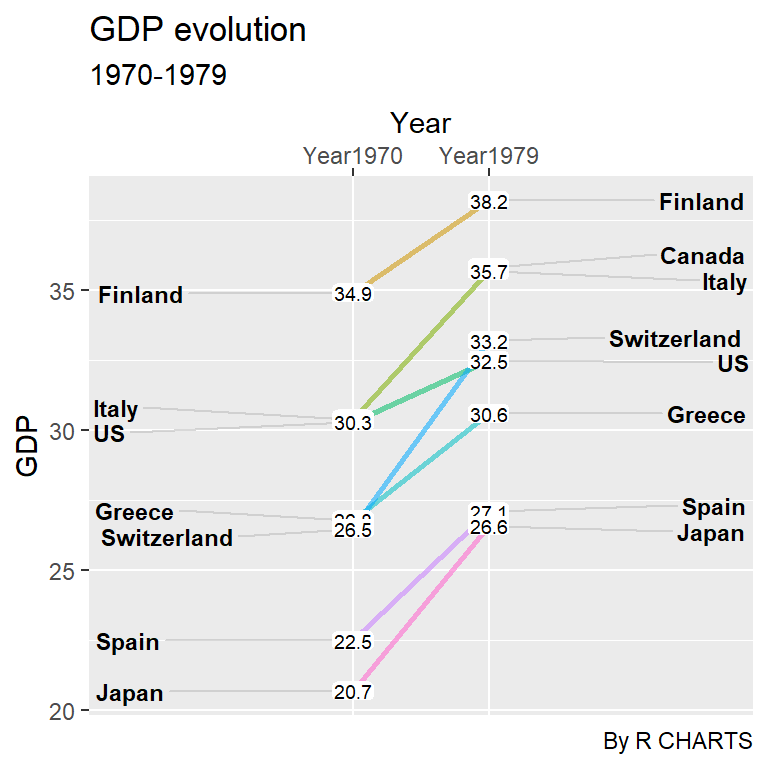# Slopegraph in ggplot2

Chuck Powell

## Sample data

Consider the following subset of the `newgdp` data frame from the `CGPfunctions` package, that also contains the function we are going to use in this tutorial.

``````# install.packages("CGPfunctions")
library(CGPfunctions)

df <- newgdp[16:30, ]``````

## The `newggslopegraph` function from the `CGPfunctions` package

Given a data frame with at least three columns that represent the times (X axis), the measurement (Y axis) and the grouping (the different groups) you can create a slope graph in ggplot2 making use of the `newggslopegraph` from `CGPfunctions` package passing the data frame and specifying the names of the columns:

``````# install.packages("CGPfunctions")
library(CGPfunctions)

newggslopegraph(dataframe = df,
Times = Year,
Measurement = GDP,
Grouping = Country)``````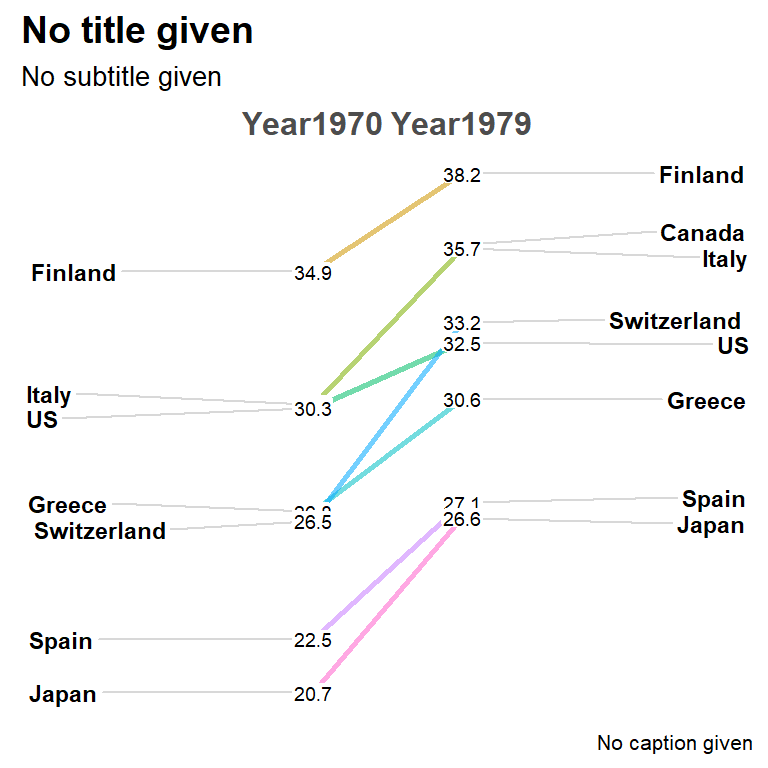The `Data.label` argument is an optional argument that can be used to pass a character column to override the plotted values. By default the column passed to `Measurement` is converted to character and used.

The function displays some titles by default. If you want to change them use the following arguments. Note that if you set them to `NULL` you can remove the titles.

``````# install.packages("CGPfunctions")
library(CGPfunctions)

newggslopegraph(df, Year, GDP, Country,
Title = "GDP evolution",
SubTitle = "1970-1979",
Caption = "By R CHARTS")``````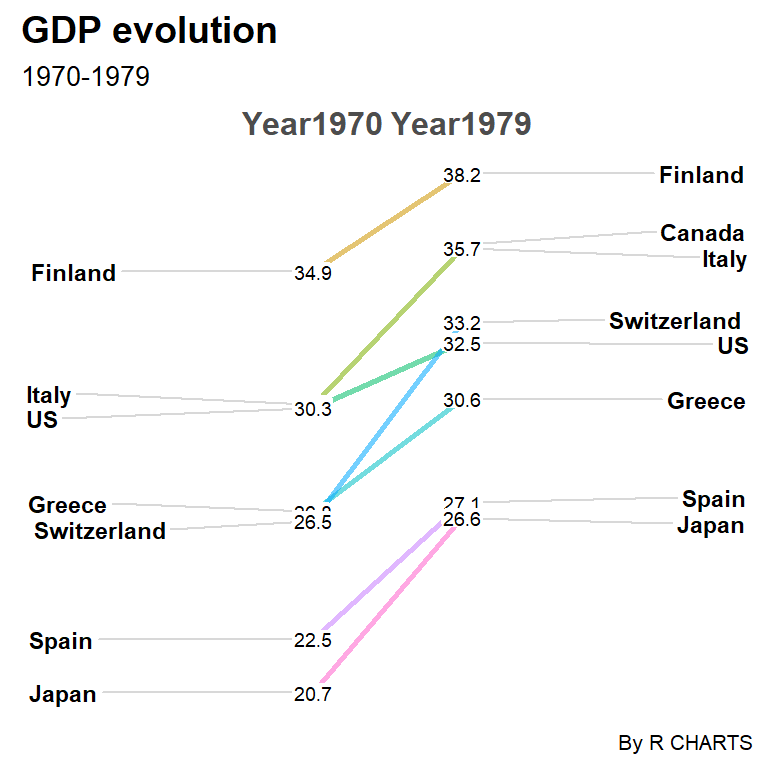Text sizes and justification

The function also provides lots of arguments to customize the size and alingment of the texts. The argument names are self explanatory.

``````# install.packages("CGPfunctions")
library(CGPfunctions)

newggslopegraph(df, Year, GDP, Country,
Title = "GDP evolution",
SubTitle = "1970-1979",
Caption = "By R CHARTS",
XTextSize = 18,    # Size of the times
YTextSize = 2,     # Size of the groups
TitleTextSize = 14,
SubTitleTextSize = 12,
CaptionTextSize = 10,
TitleJustify = "right",
SubTitleJustify = "right",
CaptionJustify = "left",
DataTextSize = 2.5) # Size of the data``````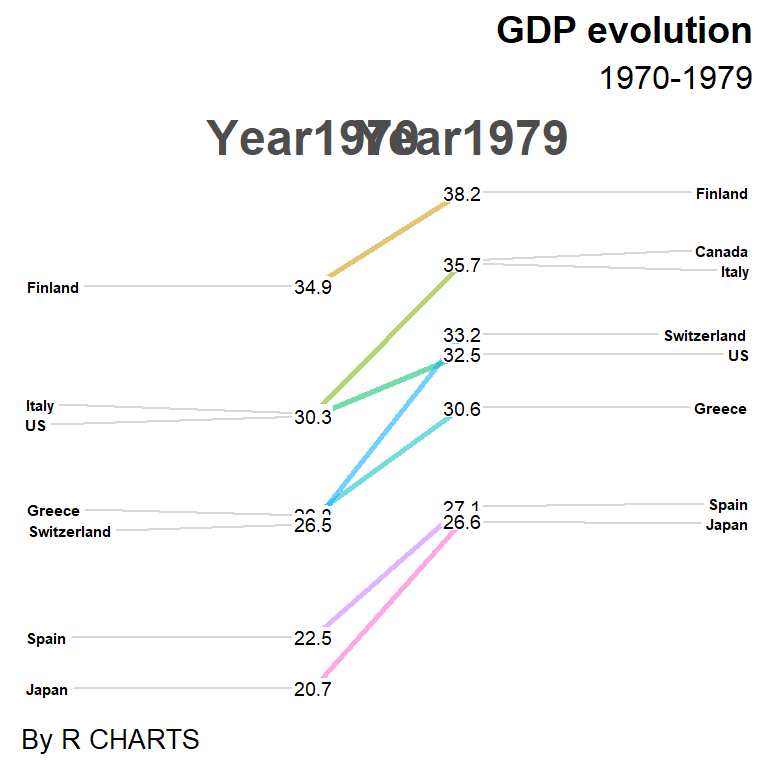Reverse axes

Note that you can also reverse the axes. Type `?newggslopegraph` for additional details.

``````# install.packages("CGPfunctions")
library(CGPfunctions)

newggslopegraph(df, Year, GDP, Country,
Title = "GDP evolution",
SubTitle = "1970-1979",
Caption = "By R CHARTS",
ReverseYAxis = TRUE,
ReverseXAxis = FALSE)      ``````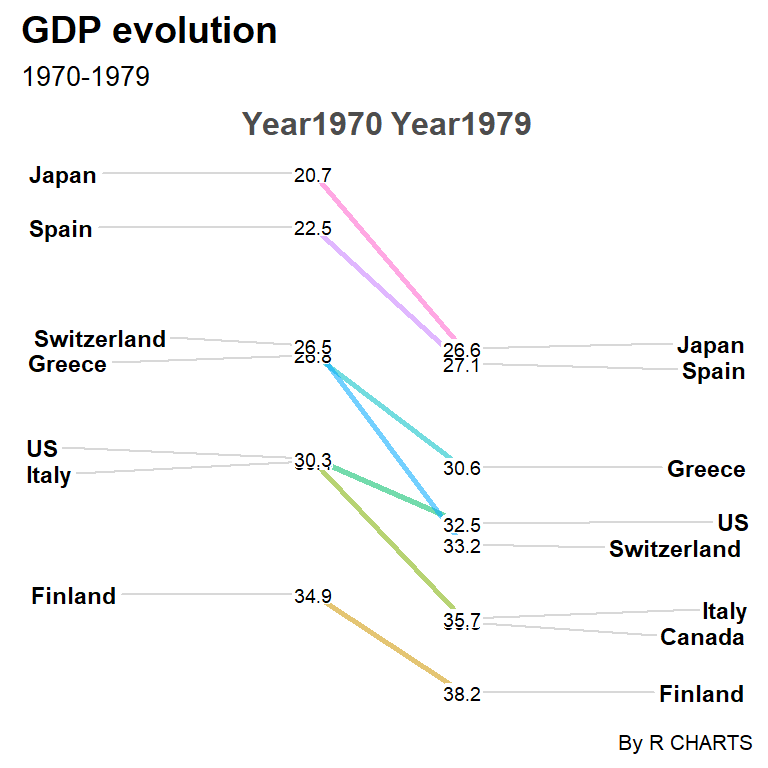## Colors and lines customization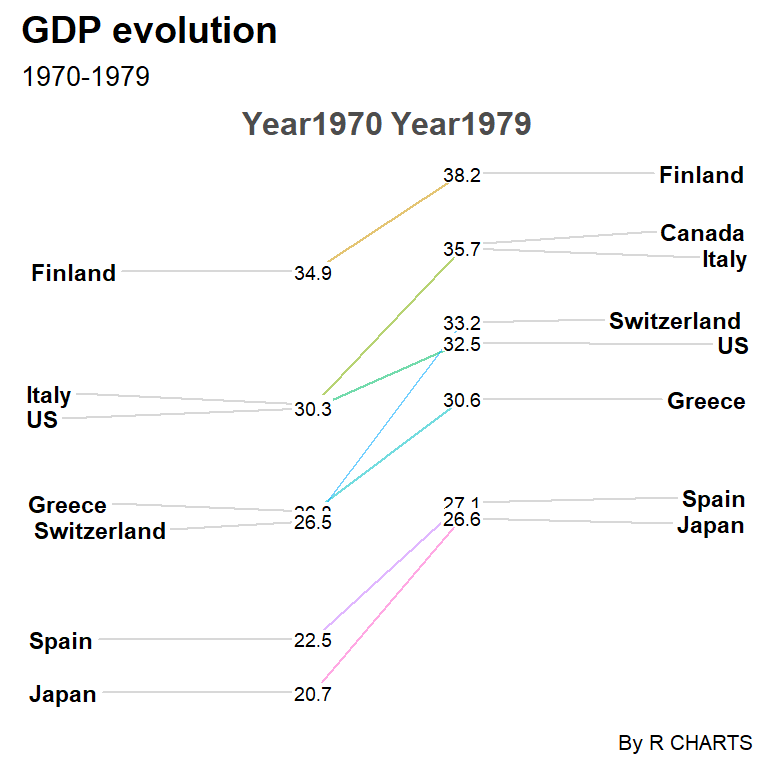Line tickness

By default, the widths of the lines of the chart are equal to 1, but you can change them with the `LineThickness` argument.

``````# install.packages("CGPfunctions")
library(CGPfunctions)

newggslopegraph(df, Year, GDP, Country,
Title = "GDP evolution",
SubTitle = "1970-1979",
Caption = "By R CHARTS",
LineThickness = 0.5)      ``````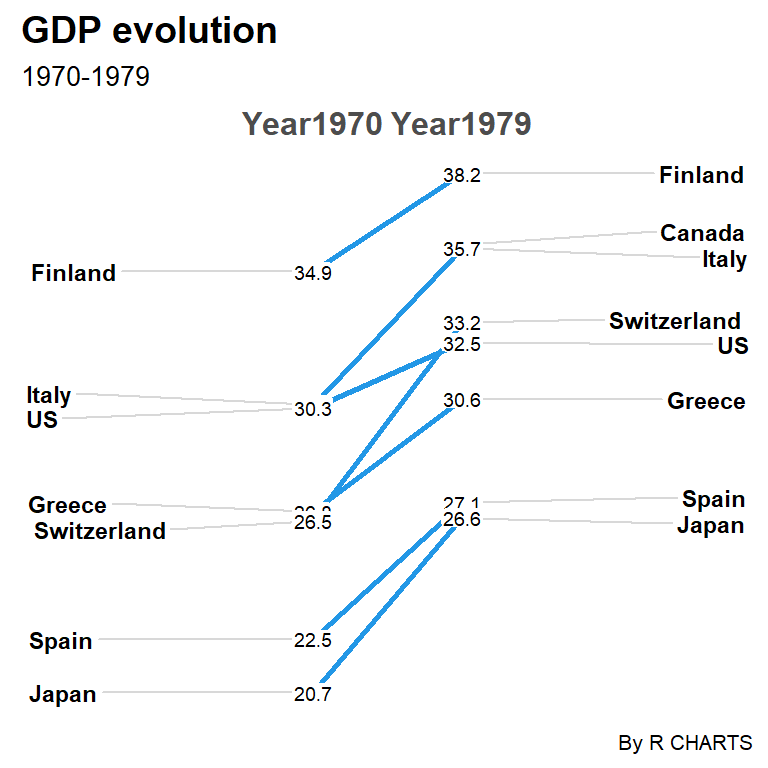Line color (one color)

The function uses the classic ggplot2 color palette by default. If you want to override this palette you can pass a color to the `LineColor` argument.

``````# install.packages("CGPfunctions")
library(CGPfunctions)

newggslopegraph(df, Year, GDP, Country,
Title = "GDP evolution",
SubTitle = "1970-1979",
Caption = "By R CHARTS",
LineColor = 4)     ``````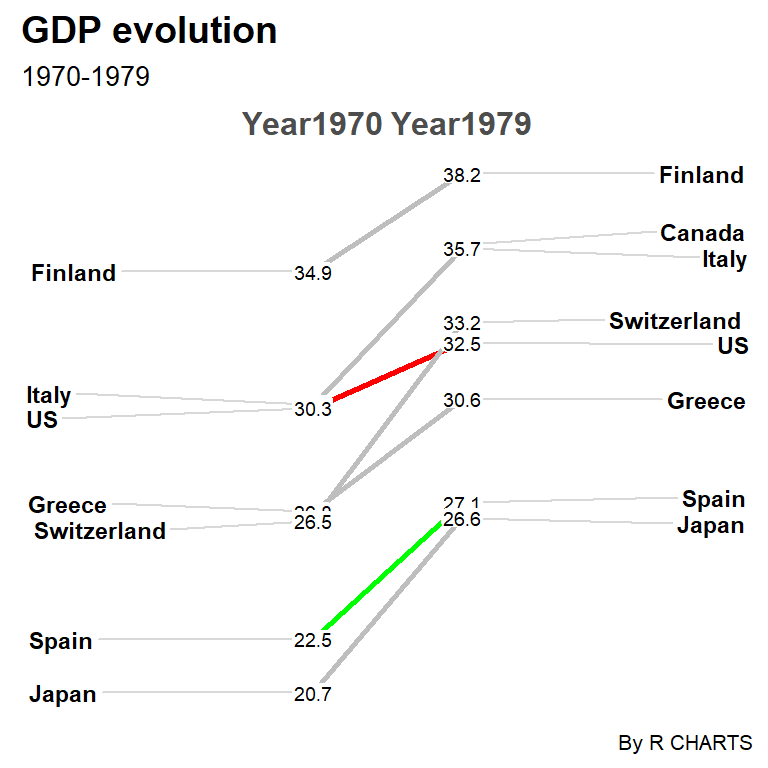Line color (several colors)

If you want to customize the color for each line you will need to create a named vector with the group names. This can be used to highlight some groups as in the example below.

``````# install.packages("CGPfunctions")
library(CGPfunctions)

cols <- c("Finland" = "gray", "Canada" = "gray",
"Italy" = "gray", "US" = "red",
"Greece" = "gray", "Switzerland" = "gray",
"Spain" = "green", "Japan" = "gray")

newggslopegraph(df, Year, GDP, Country,
Title = "GDP evolution",
SubTitle = "1970-1979",
Caption = "By R CHARTS",
LineColor = cols)     ``````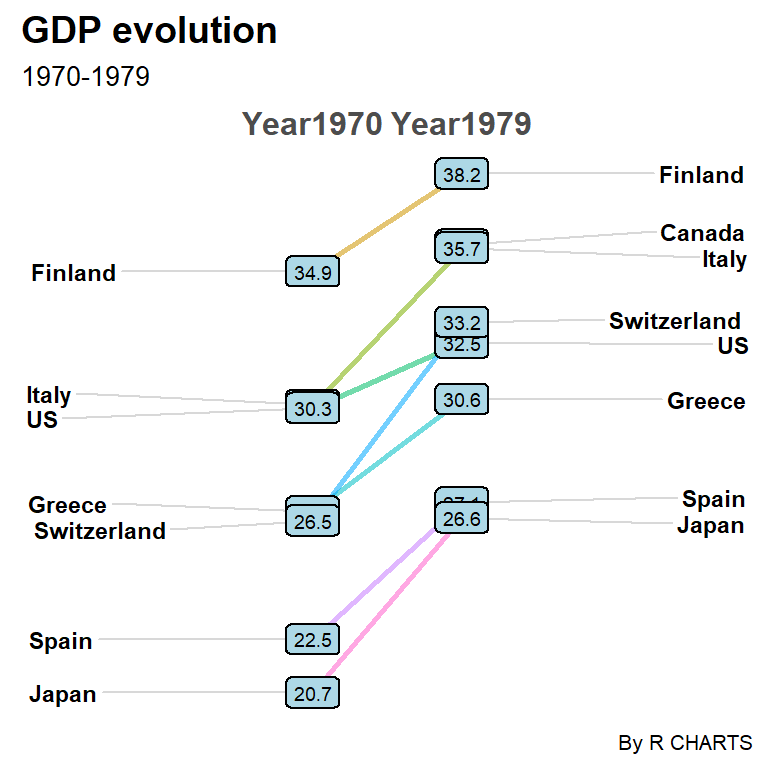Labels background color

If for some reason you want to highlight the values of the graph you can add a fill color and a border to them. As the default padding is 0 you can also increase it for better visualization.

``````# install.packages("CGPfunctions")
library(CGPfunctions)

newggslopegraph(df, Year, GDP, Country,
Title = "GDP evolution",
SubTitle = "1970-1979",
Caption = "By R CHARTS",
DataLabelLineSize = 0.5,
DataLabelFillColor = "lightblue")``````

## Themes

Finally, the function also provides the `ThemeChoice` argument to modify the theme used. The default value is `"bw"` and the possible options are displayed below.

“ipsum” theme

``````# install.packages("CGPfunctions")
library(CGPfunctions)
# install.packages("hrbrthemes")

newggslopegraph(df, Year, GDP, Country,
Title = "GDP evolution",
SubTitle = "1970-1979",
Caption = "By R CHARTS",
ThemeChoice = "ipsum")``````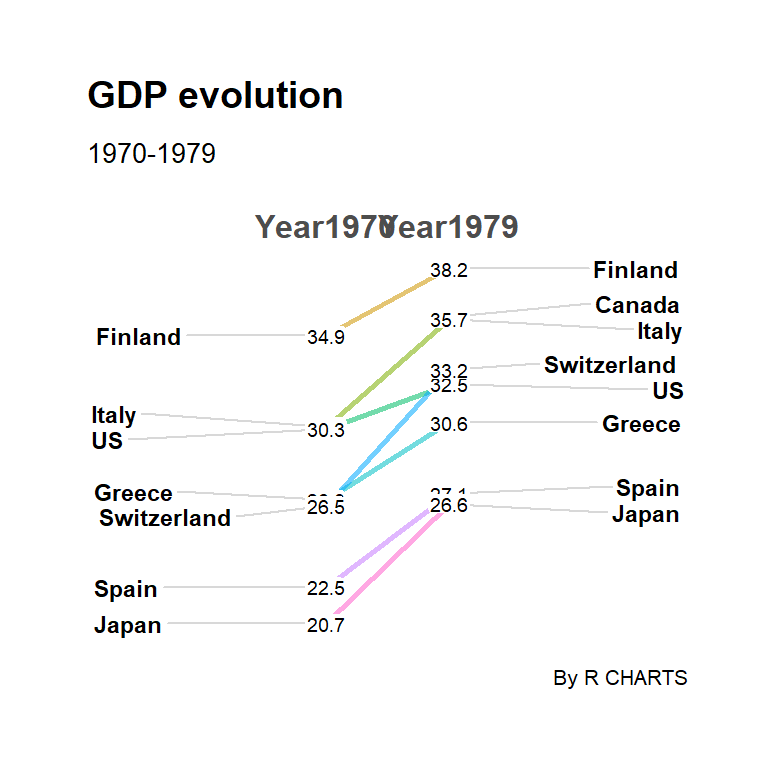“econ” theme

``````# install.packages("CGPfunctions")
library(CGPfunctions)
# install.packages("ggthemes")

newggslopegraph(df, Year, GDP, Country,
Title = "GDP evolution",
SubTitle = "1970-1979",
Caption = "By R CHARTS",
ThemeChoice = "econ")``````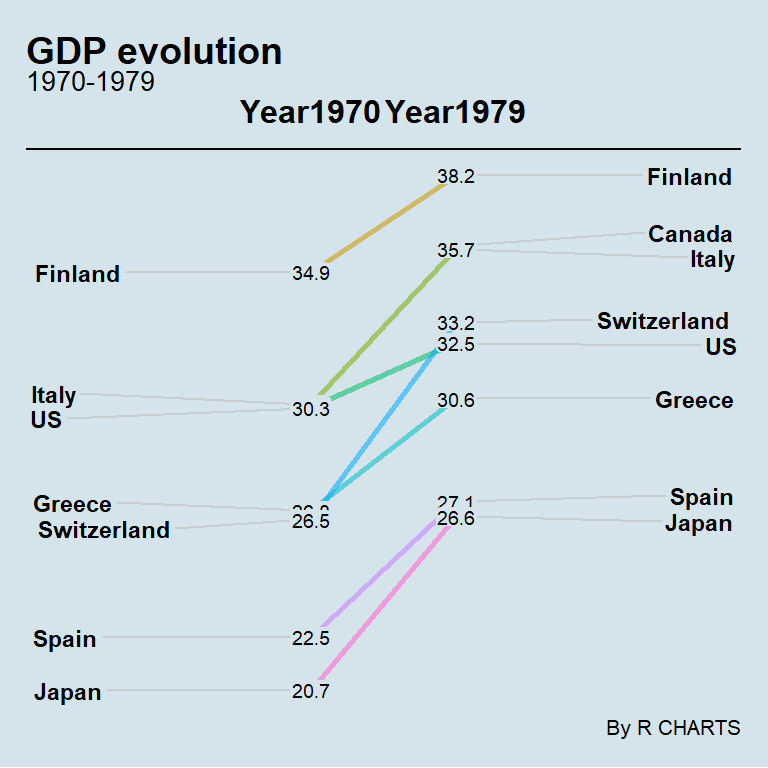“wsj” theme

``````# install.packages("CGPfunctions")
library(CGPfunctions)
# install.packages("ggthemes")

newggslopegraph(df, Year, GDP, Country,
Title = "GDP evolution",
SubTitle = "1970-1979",
Caption = "By R CHARTS",
ThemeChoice = "wsj")``````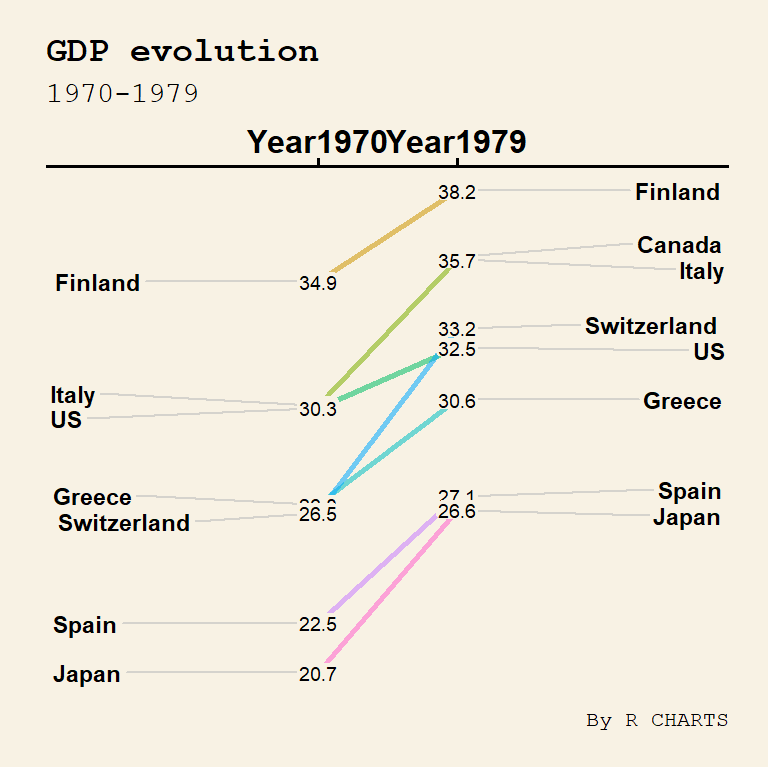“gdocs” theme

``````# install.packages("CGPfunctions")
library(CGPfunctions)
# install.packages("ggthemes")

newggslopegraph(df, Year, GDP, Country,
Title = "GDP evolution",
SubTitle = "1970-1979",
Caption = "By R CHARTS",
ThemeChoice = "gdocs")``````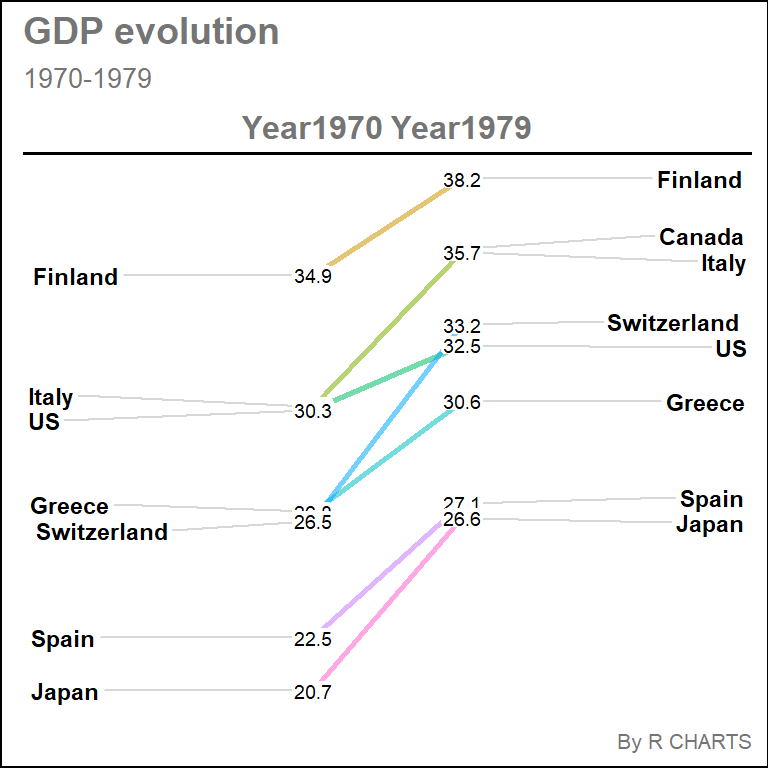“tufte” theme

``````# install.packages("CGPfunctions")
library(CGPfunctions)
# install.packages("ggthemes")

newggslopegraph(df, Year, GDP, Country,
Title = "GDP evolution",
SubTitle = "1970-1979",
Caption = "By R CHARTS",
ThemeChoice = "tufte")``````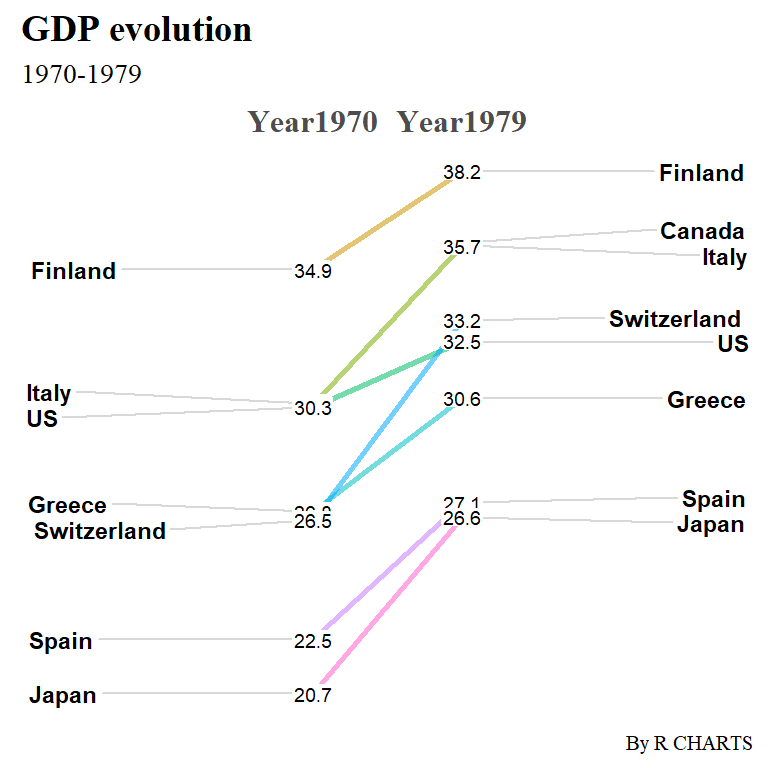Other themes

As the function is based on ggplot2 you can also add more themes adding a new layer. In the following example we are passing the default ggplot2 theme.

``````# install.packages("CGPfunctions")
library(CGPfunctions)
# install.packages("ggplot2")
library(ggplot2)

newggslopegraph(df, Year, GDP, Country,
Title = "GDP evolution",
SubTitle = "1970-1979",
Caption = "By R CHARTS") +
theme_gray() +
theme(legend.position = "none")``````